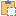#### AreaReinforcement Class

An object that represents an Area Reinforcement within the Autodesk Revit project.

Namespace: Autodesk.Revit.DB.Structure
Assembly: RevitAPI (in RevitAPI.dll) Version: 2015.0.0.0 (2015.0.0.0)

# Syntax

C#
``public class AreaReinforcement : Element``
Visual Basic
``````Public Class AreaReinforcement _
Inherits Element``````
Visual C++
``public ref class AreaReinforcement : public Element``

# Remarks

This object derived from the Element base object and such supports all the methods of that object such as the ability to retrieve the parameters of that object. The Area Reinforcement element is available only in the Autodesk Revit Structure product.

# ExamplesCopy C#
``````public void GetInfo_AreaReinforcement(AreaReinforcement areaReinforcement)
{
string message = "AreaReinforcement : ";

// Show the AreaReinforcement Type information
//message += "\nType : " + areaReinforcement.AreaReinforcementType.Name;

// Show the AreaReinforcement bar information
IList<ElementId> rebarInSystemIds = areaReinforcement.GetRebarInSystemIds();
message += "\nNumber of distinct bar shapes : " + rebarInSystemIds.Count;

for (int i = 0; i < rebarInSystemIds.Count; i++)
{
RebarInSystem ris = doc.GetElement(rebarInSystemIds) as RebarInSystem;
message += "\nBar count : " + ris.Quantity;
message += "\nBar type name : " + ris.Name;
message += "\nBar length : " + ris.LookupParameter("Bar Length").AsDouble();
}

// Show the AreaReinforcement Curves information
IList<ElementId> curveIds = areaReinforcement.GetBoundaryCurveIds();
message += "\nArea Reinforcement has " + curveIds.Count + " boundary curves.";
foreach (Autodesk.Revit.DB.ElementId ii in curveIds)
{
AreaReinforcementCurve reinCurve = doc.GetElement(ii) as AreaReinforcementCurve;
if (null == reinCurve)
{
continue;
}
Curve curve = reinCurve.Curve; // get the location curve
XYZ start = curve.GetEndPoint(0);  // get the start point of the curve
XYZ end = curve.GetEndPoint(1);    // get the end point of the curve
message += "\nCurve: Start point (" + start.X + ", " + start.Y + ", " + start.Z + ")";
message += "\n       End point (" + end.X + ", " + end.Y + ", " + end.Z + ")";
}

}``````Copy VB.NET
``````Public Sub GetInfo_AreaReinforcement(areaReinforcement As AreaReinforcement)
Dim message As String = "AreaReinforcement : "

' Show the AreaReinforcement Type information
'message += "\nType : " + areaReinforcement.AreaReinforcementType.Name;

' Show the AreaReinforcement bar information
Dim rebarInSystemIds As IList(Of ElementId) = areaReinforcement.GetRebarInSystemIds()
message += vbLf & "Number of distinct bar shapes : " & rebarInSystemIds.Count

For i As Integer = 0 To rebarInSystemIds.Count - 1
Dim ris As RebarInSystem = TryCast(doc.GetElement(rebarInSystemIds(0)), RebarInSystem)
message += vbLf & "Bar count : " + ris.Quantity
message += vbLf & "Bar type name : " + ris.Name
message += vbLf & "Bar length : " & ris.LookupParameter("Bar Length").AsDouble()
Next

' Show the AreaReinforcement Curves information
Dim curveIds As IList(Of ElementId) = areaReinforcement.GetBoundaryCurveIds()
message += vbLf & "Area Reinforcement has " & curveIds.Count & " boundary curves."
For Each ii As Autodesk.Revit.DB.ElementId In curveIds
Dim reinCurve As AreaReinforcementCurve = TryCast(doc.GetElement(ii), AreaReinforcementCurve)
If reinCurve Is Nothing Then
Continue For
End If
Dim curve As Curve = reinCurve.Curve
' get the location curve
Dim start As XYZ = curve.GetEndPoint(0)
' get the start point of the curve
Dim [end] As XYZ = curve.GetEndPoint(1)
' get the end point of the curve
message += ((vbLf & "Curve: Start point (" + start.X & ", ") + start.Y & ", ") + start.Z & ")"
message += ((vbLf & "       End point (" + [end].X & ", ") + [end].Y & ", ") + [end].Z & ")"
Next